## Introduction

It’s Rishab’s birthday. He has 5 best friends. He wants to give his friends chocolates in such a way that:

• 1st friend gets 2 chocolates
• 2nd friend gets double the number of chocolates the 1st friend gets, i.e.
2 × 2 = 4
• 3rd friend gets double the number of chocolates the 2nd friend gets, i.e.
4 × 2 = 8
• 4th friend gets double the number of chocolates the 3rd friend gets, i.e.
8 × 2 = 16
• 5th friend gets double the number of chocolates the 4th friend gets, i.e.
16 × 2 = 32

Observe the table: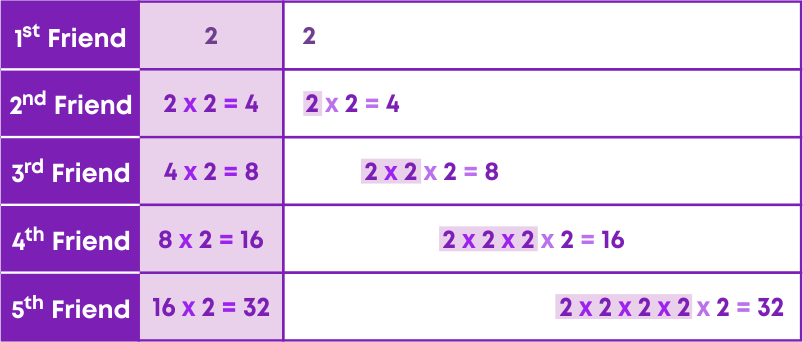Note: double = two times

Just like how repeated addition is expressed as multiplication, repeated multiplication is expressed as POWERS.

Let's watch a video to know a little more about exponents and powers.

In this video, we saw

• how exponential form of numbers can be used to easily represent, understand, compare and compute numbers.
• how exponential numbers are used in real-life applications.

Do you remember studying mensuration in your previous classes? How did you recall the formula and units for the area? The area is calculated by squaring two sides, and the unit is written as a square. The square here is nothing but power. All units of area are written as squares.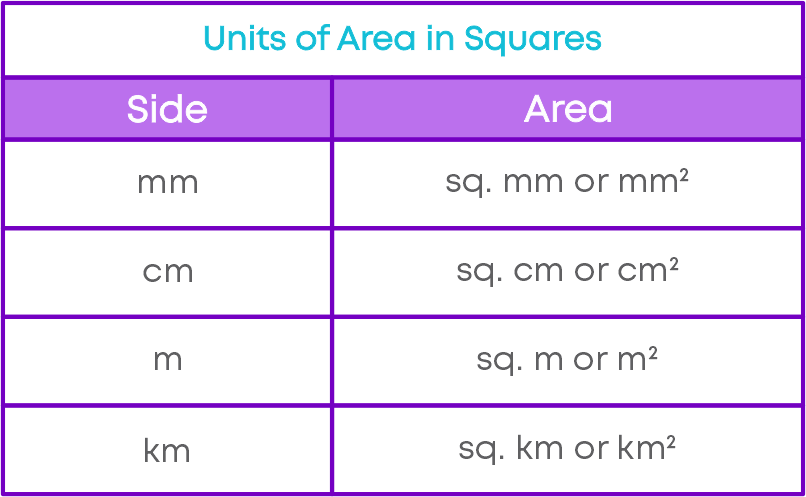#### Concepts

The chapter ‘Exponents and Powers’ covers the following concepts:

#### Exponents

Numbers can be expressed in different forms: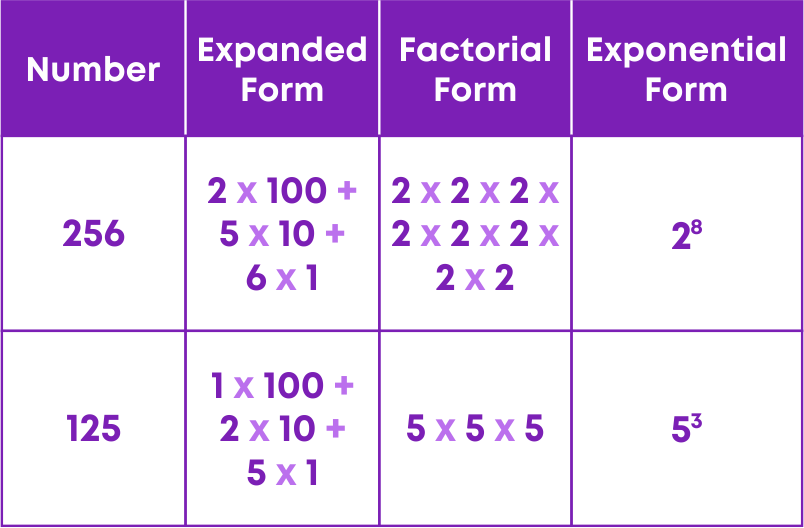Exponential form is an easier way to write a number as a product of many factors. Exponential form can be used to easily represent, understand, compare and compute large numbers.

We have learnt that the exponential form is an easier way to write a large number as a product of many factors.

Consider squares and cubes of a number.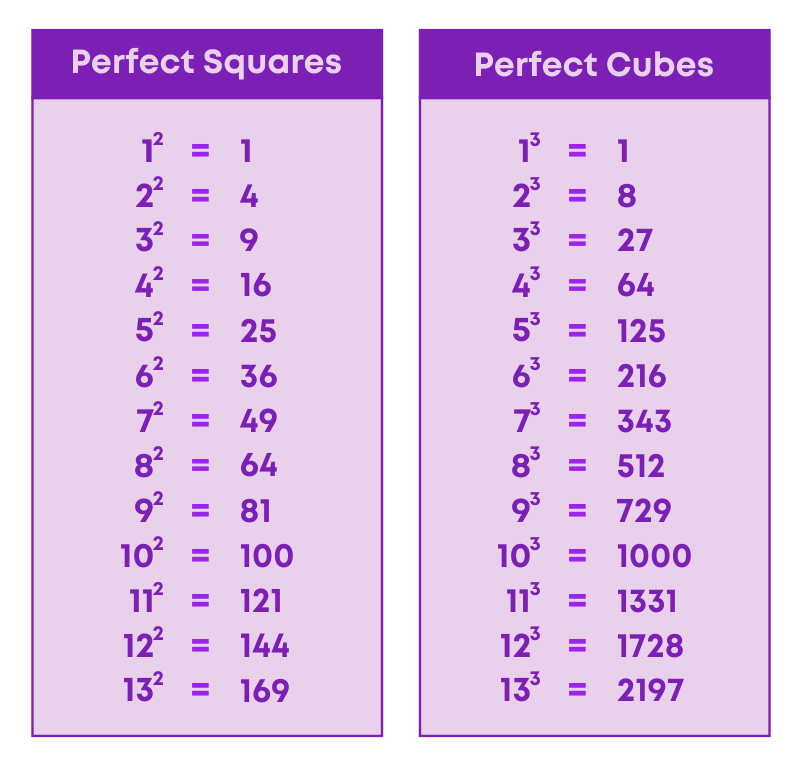In both the contexts, a larger number is expressed in terms of a smaller number raised to a certain power.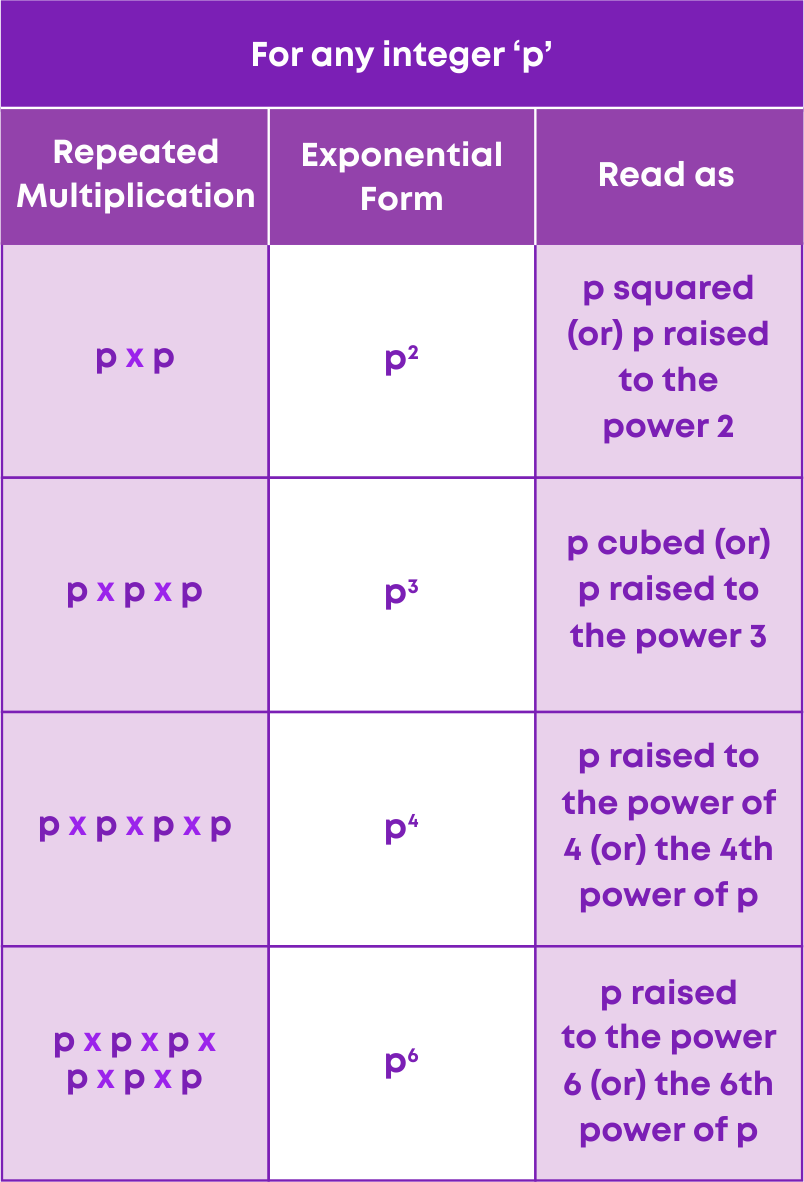Therefore, for any non-zero integer ‘a’ and a whole number ‘m’, the generalized exponential form is am,

Where ‘a’ is the base and ‘m’ is the exponent.

• Base – Base is the number that undergoes repeated multiplication.
• Exponent – An exponent corresponds to the number of times the base is used as a factor.

Examples:

• 5 × 5 × 5 × 5 × 5 = 55, i.e., the repeated multiplication of 5 can be expressed in exponential form.
• The exponential form 124 can be written in the repeated multiplication form, i.e., 124 = 12 × 12 × 12 × 12.

We know that 1 raised to the power of any number gives us 1. But, will -1 raised to the power of any number still give 1? No!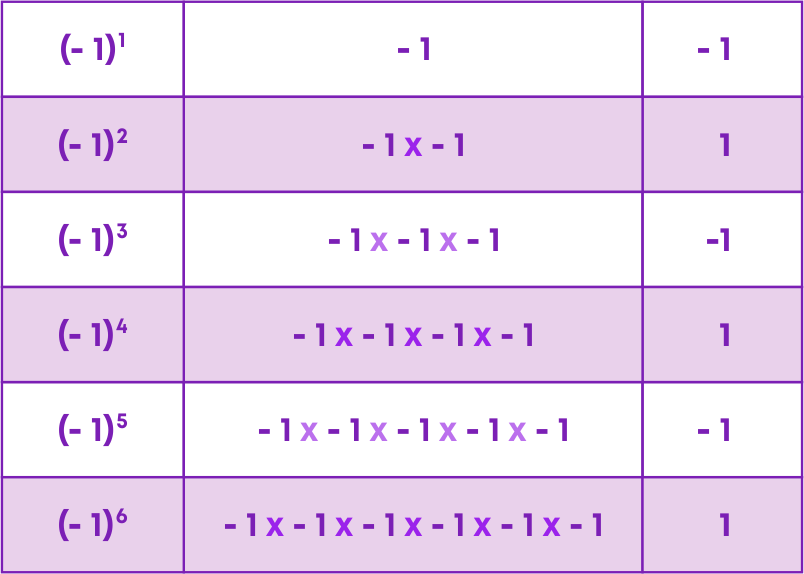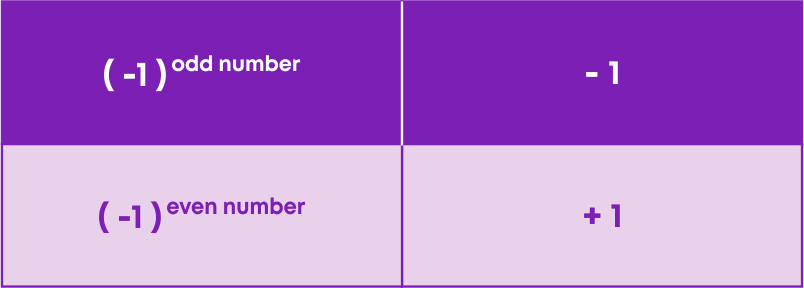Therefore, any negative number raised to the power of an even number gives a positive number, and if it is raised to the power of an odd number, it results in a negative number.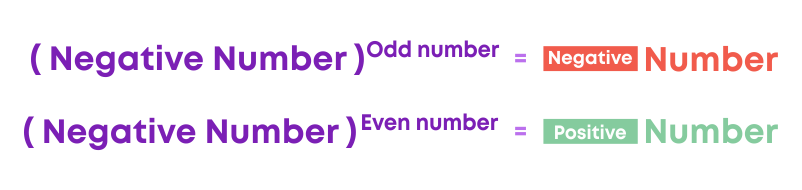Examples:

• (-3)3 = -3 × -3 × -3 = -27, a negative number
• On the contrary, (-3)4 = -3 × -3 × -3 × -3 = 81, a positive number

We know that the square of a number ‘p’ is written as p2 = p × p, and the cube of a number ‘r’ is written as r3 = r × r × r.

Thus, the value of a number raised to any power – which is in the exponential form, can be determined by breaking it down into its factors.

If ‘a’ and ‘b’ are two integers, then the value ofFor example, 42 × 53 = 4 × 4 × 5 × 5 × 5 = 16 × 125 = 2000.

Comparing numbers with the same base is easy. For example, 44 < 45. Now let us see how to compare numbers with different bases and different exponents. Let’s compare 45 and 54.

45 = 4 × 4 × 4 × 4 × 4 = 1024

54 = 5 × 5 × 5 × 5 = 625

1024 > 625

Which implies 45 > 54

Note: don’t get deceived by the base value.

Do you know how to order the exponential form of numbers? By finding the value of the numbers in the exponential form, we can arrange the exponential form of numbers in ascending or descending order.

For example, arrange 23, 33, 42 in ascending order.

23 = 2 × 2 × 2 = 8

33 = 3 × 3 × 3 = 27

42 = 4 × 4 = 16

8 < 16 < 27

Therefore, 23 < 42 < 33

To express numbers as the product of powers of their prime factors, we need to know how to perform prime factorization.

Consider the number 30.Perform prime factorization: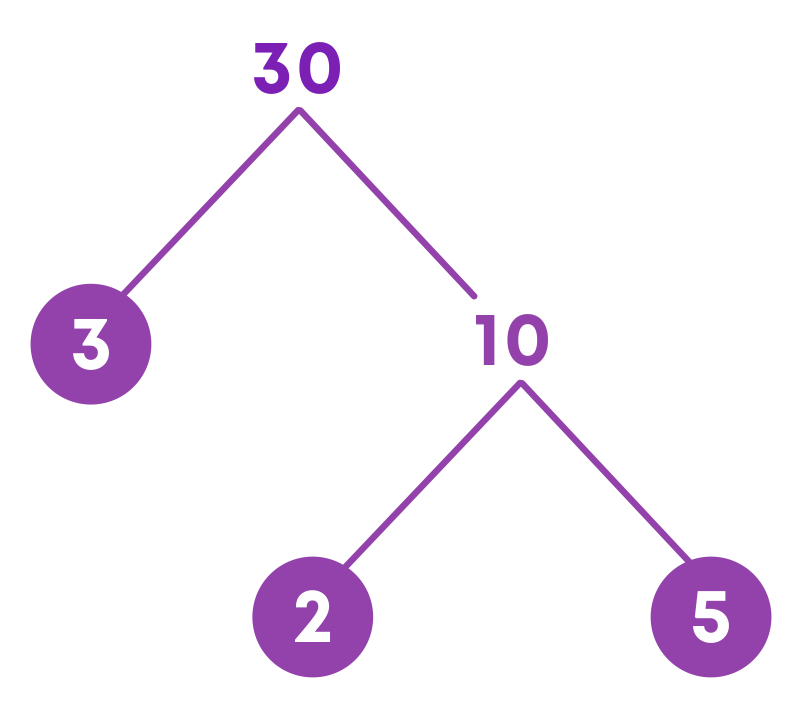We get 30 = 2 × 3 × 5.

We can express any positive integer as the product of powers of its prime factors.

Can every number be expressed in exponential form? Yes. Is it necessary to express all the numbers in exponential form? No.

So far, we have learnt about exponential form of numbers and how to express different numbers as powers or product of powers of their prime factors.

However, consider the number 33.

33 can be expressed as 33 × 100 or 31 × 111

Both are the exponential forms of 33. But, it isn’t essentially necessary to express 33 in exponential form. Similarly, numbers like 10, 15, 55, 119, 133, etc. need not be expressed in the exponential form.

The exponential form is used to handle very large or very small numbers.

We expand the powers into repeated multiplication, simplify and solve expressions involving powers. We use the BODMAS rule to simplify expressions involving powers with many operations.

#### Laws of Exponents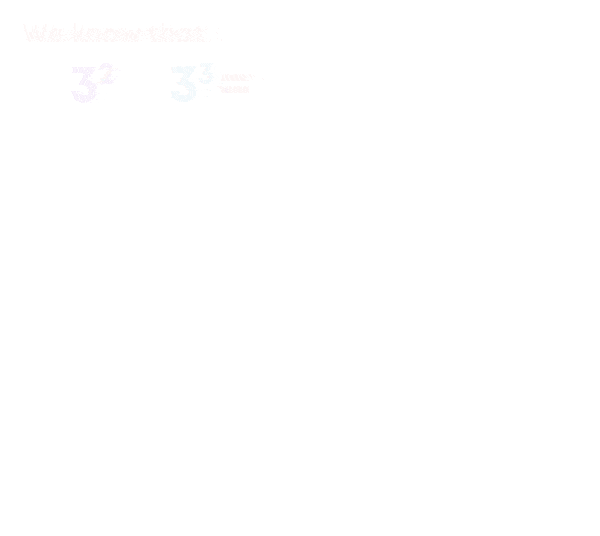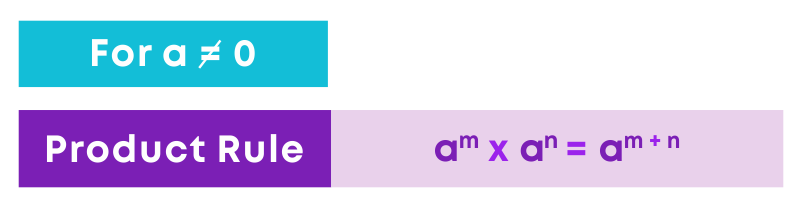Let us solve some more examples:

• 465 × 4620 = 4625
• b20 × b12 × b8 = b20+12+8 = b40

When the product of two or more powers has the same base with varying/same exponents, we can add the exponents of the base.

Using the following strategy, we can solve problems involving powers.

• Express all numbers as a power of their prime factors
• Group the numbers with the same base
• Identify the appropriate laws of exponents
• Simplify.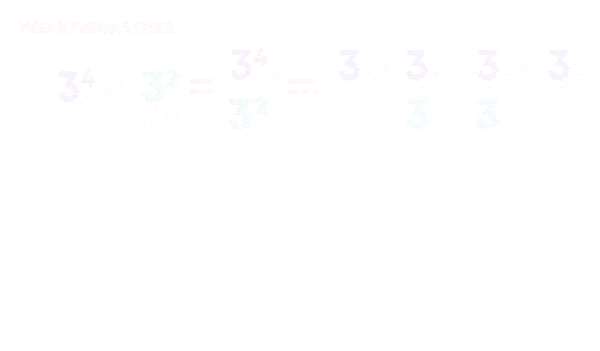To find the value of unknown exponents, we follow the strategy as given below.

• Evaluate the expression on the LHS.
• Equate the exponents on LHS and RHS.
• Solve the equation and find the result.While taking the power of an exponential number, i.e., power of a power, we simply multiply the powers.

Now consider:Now let us solve 74 ÷ 54

We write it as 74 / 54 = 7 ×7 ×7 ×7 / 5 ×5 ×5 ×5 = (7/5)4

Now consider:Any non-zero number raised to the exponent ‘0’ is equal to 1.

Let us quickly recall all the law of exponents:

For any non-zero integers ‘a’ and ‘b’ and, whole numbers ‘m’ and ‘n’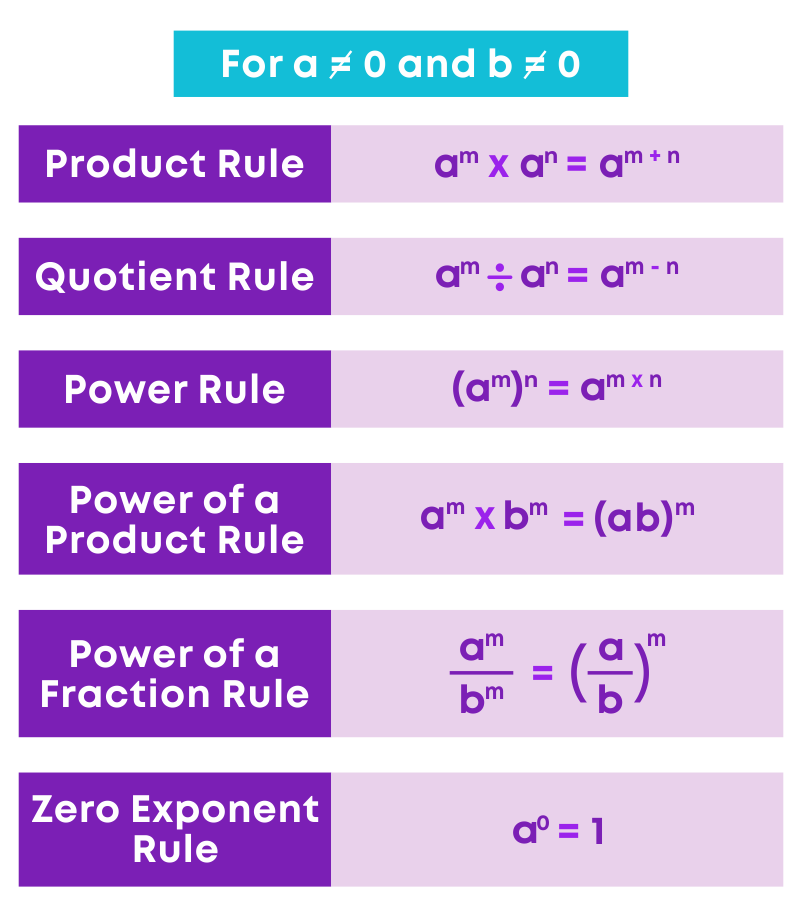#### Decimal Number System

Consider the integer 78,392. We can express it in the expanded form as

78392 = 7 × 10000 + 8 × 1000 + 3 × 100 + 9 × 10 + 2 × 1

But, we know 10000 = 104, 1000 = 103, 100 = 102, 10 = 101, 1 = 100

Therefore, we can write 78392 as 7 × 104 + 8 × 103 + 3 × 102 + 9 × 101 + 2 × 100

Let us look at another example.Observe how the exponents of 10 start from a maximum value of 8 go on decreasing by 1 at a step from the left to the right up to ‘0’.

Conversely, we can identify a number when the expanded form of the number is given.

Let us see an example.#### Expressing Large Numbers in The Standard Form

Any number can be expressed as a decimal number between 1.0 and 10.0 including 1.0 multiplied by a power of 10. Such a form of a number is called its standard form.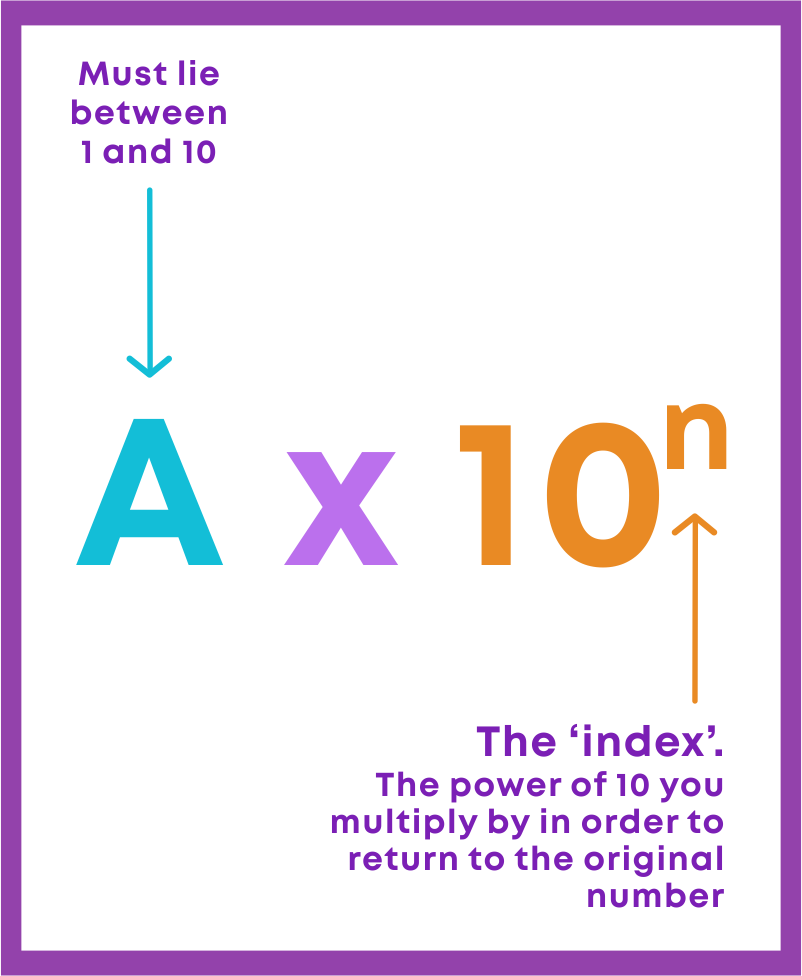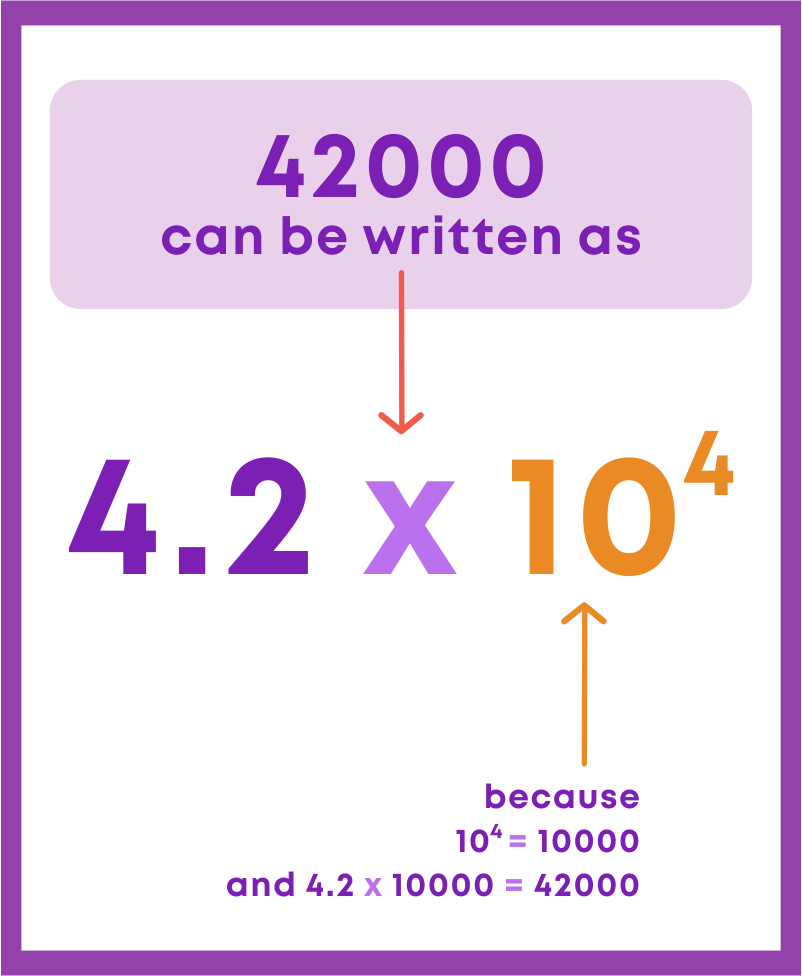Now, let us look at how large numbers can be expressed in the standard form.The mass of Earth = 5,976,000,000,000,000,000,000,000 kg.

The standard forms of the mass of Earth is as follows: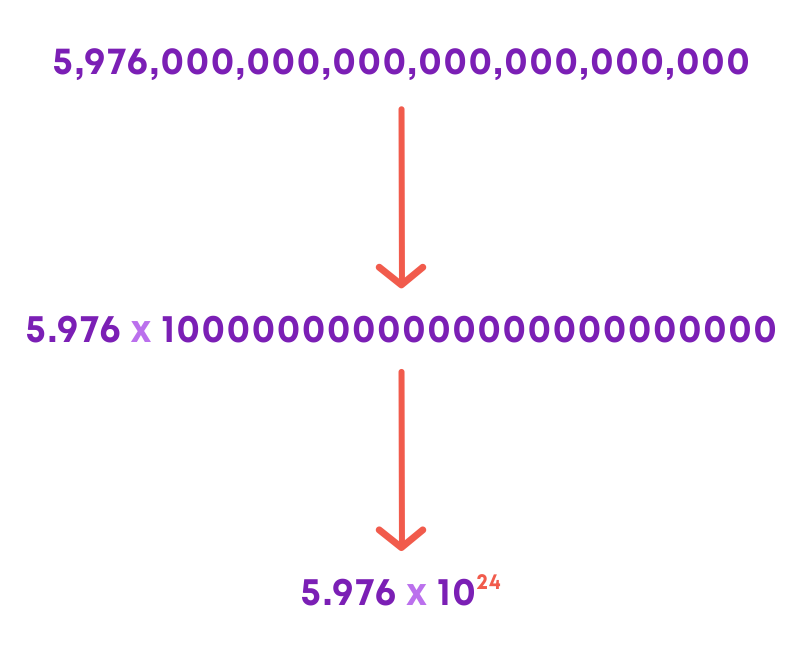The standard form is a scientific notation which is used to express very large or very small numbers.

We just learnt how to convert any number to its standard form. Now, let’s see how to convert the standard form of a number to its usual form.

Convert 4.397 × 103 in the usual form.

In the usual form, we do not have the exponents, therefore, we can write,

4.397 × 103 = 4.397 × 1000 = 4397, which is its usual form.

Now let’s see how to write the numbers in the standard form when a statement is given

In a galaxy, there are on an average 100,000,000,000 stars.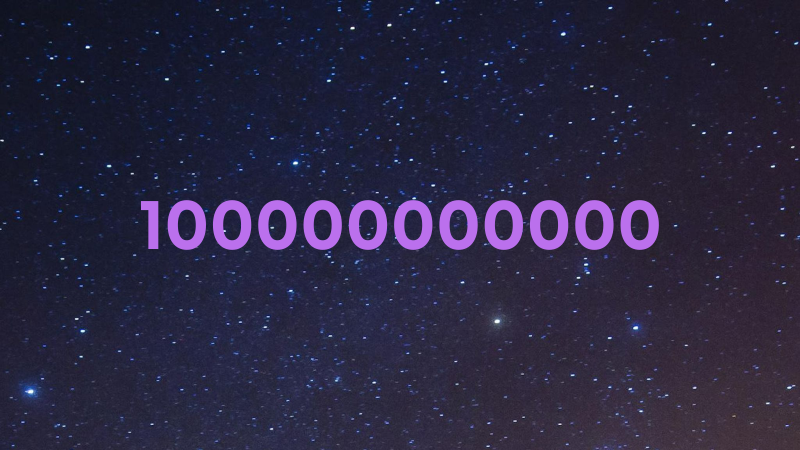100,000,000,000=1 × 100000000000

= 1 × 1011 stars in the standard form.

Now let’s learn how to compare two numbers written in the standard form.

Compare 7.5 × 107 and 3.2 × 1011

To compare two numbers that are expressed in the standard form, it is sufficient to compare their powers of 10, i.e.,

Consider 107 and 1011

11 > 7

This implies 1011 > 107

Therefore, 3.2 × 1011 > 7.5 × 107

#### Common Errors

The following are topics in which students make common mistakes when dealing with exponents and powers:

• 1. 112 is not the same as (2 × 11)
• 2. -32 is not the same as (-3)2
• 3. 4-2 is not the same as -42
• 4. 32 is not the same as 23
• 5. Expanded form of numbers
• 6. 65 × 620 is not equal to 6100
• 7. 7. 20 is not 0

#### 112 Is Not The Same As (2 × 11)

The expression 112 means to multiply 11 by itself twice, not 11 multiplied by 2.

112 = 11 × 11 = 121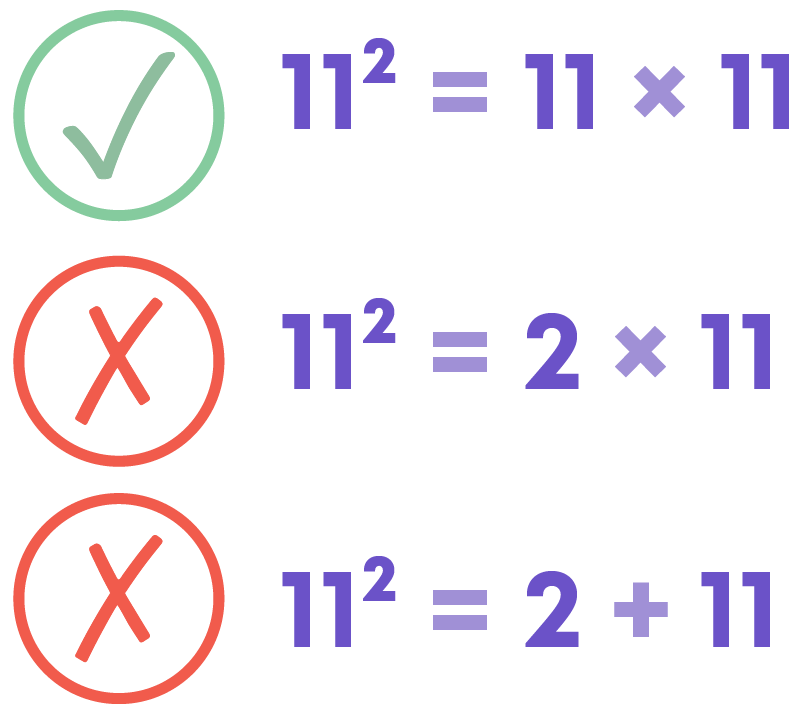#### -32 Is Not The Same As (-3)2

The expression -32 means to multiply two 3's together first (by order of operations), and then take the negative of that answer.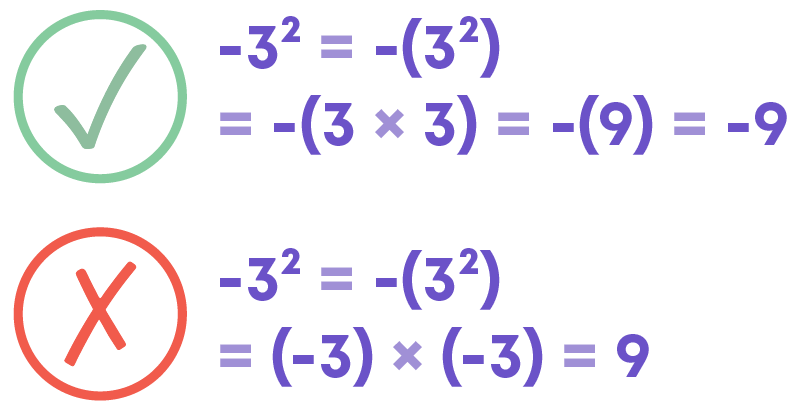The expression (-3)2 means to multiply two (-3)'s together.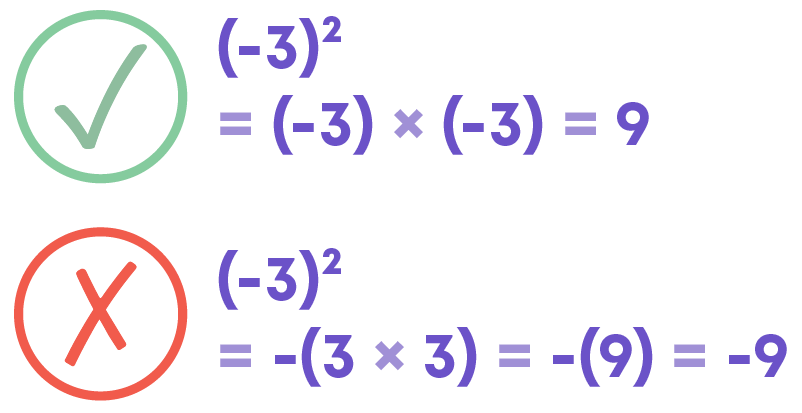#### 4-2 Is Not The Same As -42

42 means multiplication by 4 two times.

42 = 4 × 4 = 16

In the same manner, 4-2 means division by 4 two times.

​4-2 = (1/4)2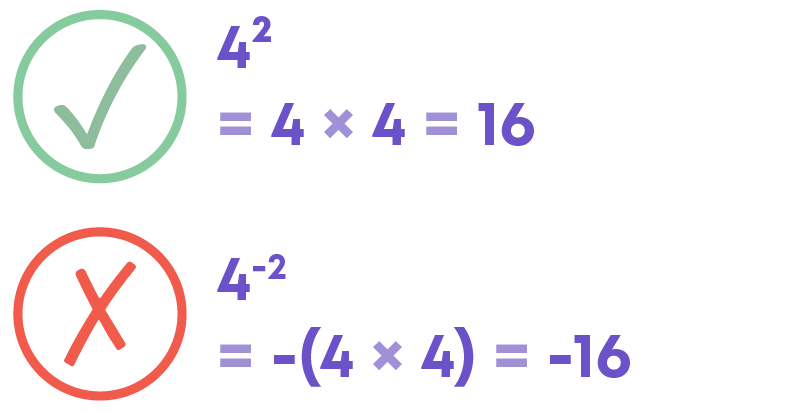#### 32 Is Not The Same As 23

32 means multiplication by 3 two times.

32 = 3 × 3 = 9

In the same manner, 23 means multiplication by 2 three times.

​23 = 2 × 2 × 2 = 8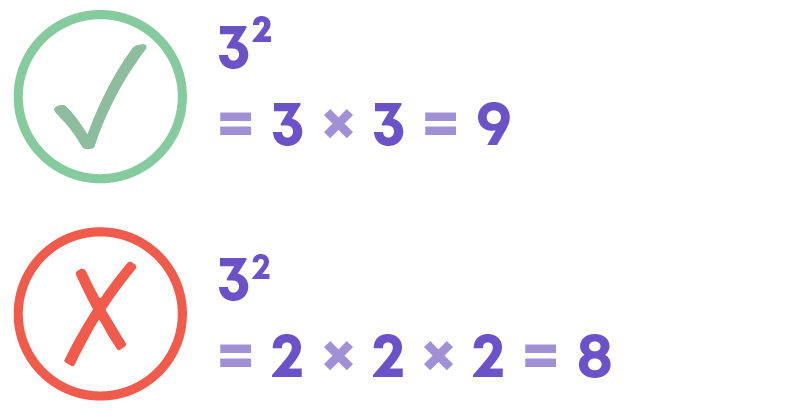#### Expanded Form Of Numbers

How will you express 256 in the expanded form? The given number is 256.

As 2 is in the hundreds place and the third number from the right, its place value is 2 × 100 and not 2 × 1000. Similarly, 5 is in the tens place and the second number from the right, so its place value is 5 × 10 and not 5 × 100.

Also, the place value of 6 is 6 × 1 and not 6 × 10.

Hence, the expanded form is 2 × 100 + 5 × 10 + 6 × 1

not 2 × 1000 + 5 × 100 + 6 × 10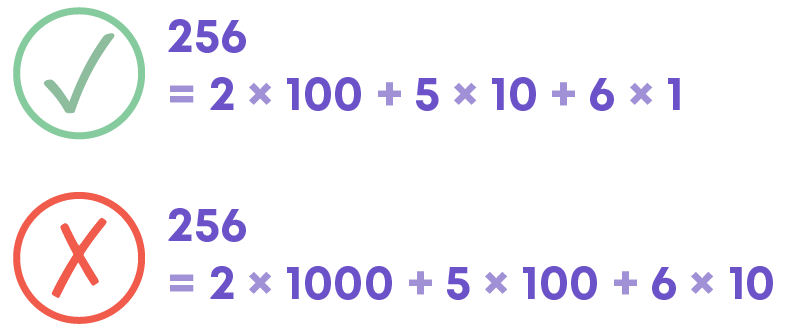#### 65 × 620 Is Not Equal To 6100

For an integer k, kp × kq × kr = kp + q + r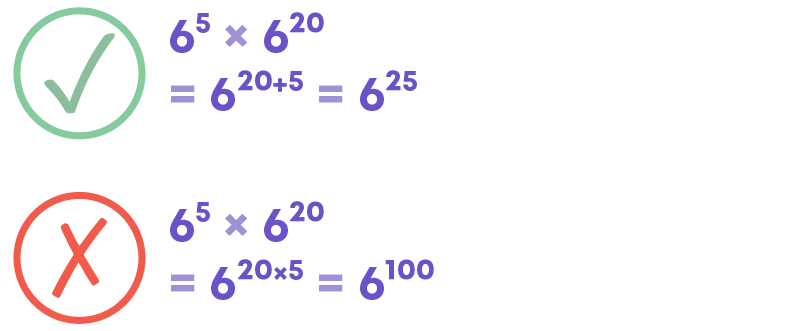#### 20 Is Not 0

Any non-zero number raised to the exponent ‘0’ is equal to 1, i.e., a0 = 1, where ‘a’ is a non-zero integer. So, 20 = 1.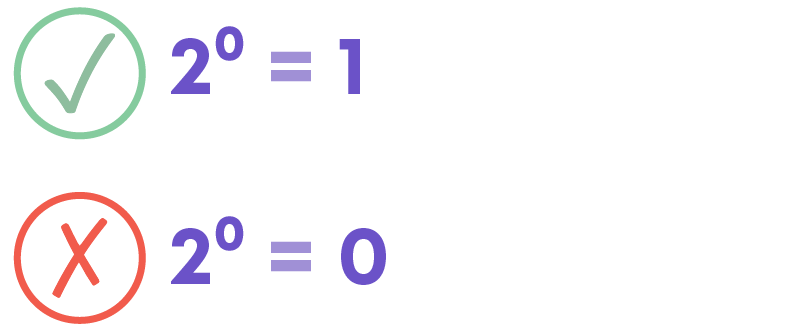#### Conclusion

Can you solve this riddle after learning all about exponents and powers?

What is the value of A and B in the given image?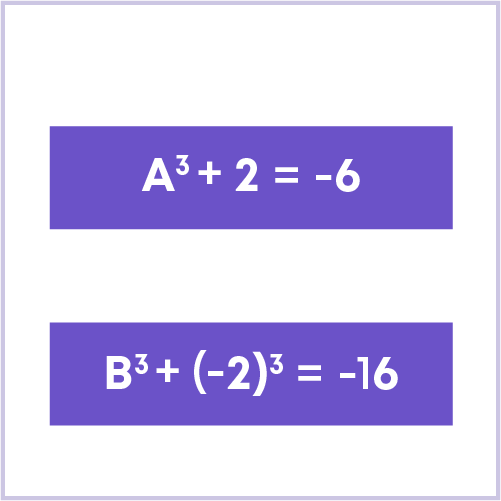#### Jyothi

Author

A math enthusiast and an educationist, Jyothi has a strong academic background and has demonstrated an impressive history of working in the education industry. Jyothi is skilled in developing and reviewing lesson plans, learning content, assessments, and video story boards and scripts.